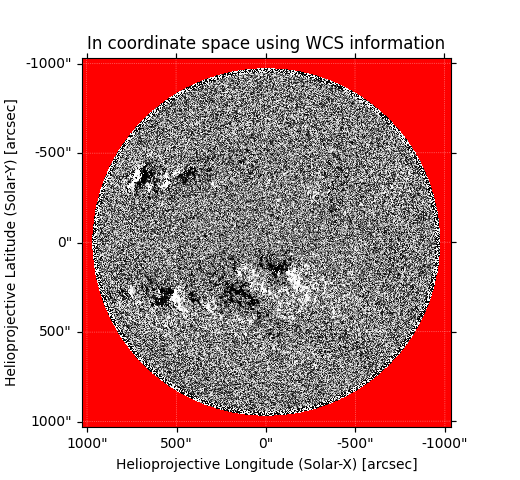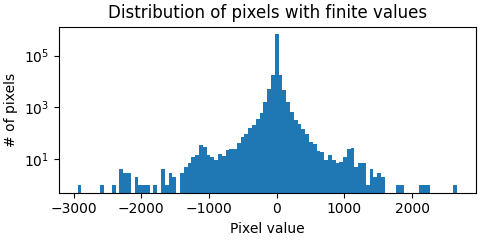# MapSequence¶

class `sunpy.map.``MapSequence`(*args, sortby='date', derotate=False, **kwargs)[source]

Bases: `object`

A series of Maps in a single object.

Parameters
• args (`list`) – A list of Map instances

• sortby ({ “date” | `None`}) – Method by which the MapSequence should be sorted along the z-axis. Defaults to sorting by: “date” and is the only supported sorting strategy. Passing `None` will disable sorting.

• derotate (`bool`) – Apply a derotation to the data. Default to False.

`maps`

This attribute holds the list of Map instances obtained from parameter args.

Type

`list`

Notes

To coalign a mapsequence so that solar features remain on the same pixels, please see the “Coalignment of MapSequences” note below.

Examples

```>>> import sunpy.map
>>> mapsequence = sunpy.map.Map('images/*.fits', sequence=True)
```

MapSequences can be co-aligned using the routines in sunpy.image.coalignment.

Methods Summary

 Tests if all the maps have the same number pixels in the x and y directions. Return all the meta objects as a list. If all the map shapes are the same, their image data is rendered into the appropriate numpy object. Tests if at least one map has a mask. `peek`([resample]) A animation plotting routine that animates each element in the MapSequence `plot`([axes, resample, annotate, interval, …]) A animation plotting routine that animates each element in the MapSequence Display a quicklook summary of the MapSequence instance using the default web browser.

Methods Documentation

`all_maps_same_shape`()[source]

Tests if all the maps have the same number pixels in the x and y directions.

`all_meta`()[source]

Return all the meta objects as a list.

`as_array`()[source]

If all the map shapes are the same, their image data is rendered into the appropriate numpy object. If none of the maps have masks, then the data is returned as a (ny, nx, nt) ndarray. If all the maps have masks, then the data is returned as a (ny, nx, nt) masked array with all the masks copied from each map. If only some of the maps have masked then the data is returned as a (ny, nx, nt) masked array, with masks copied from maps as appropriately; maps that do not have a mask are supplied with a mask that is full of False entries. If all the map shapes are not the same, a ValueError is thrown.

`at_least_one_map_has_mask`()[source]

Tests if at least one map has a mask.

`peek`(resample=None, **kwargs)[source]

A animation plotting routine that animates each element in the MapSequence

Parameters
• fig (mpl.figure) – Figure to use to create the explorer

• resample (list or False) – Draws the map at a lower resolution to increase the speed of animation. Specify a list as a fraction i.e. [0.25, 0.25] to plot at 1/4 resolution. [Note: this will only work where the map arrays are the same size]

• annotate (bool) – Annotate the figure with scale and titles

• interval (int) – Animation interval in ms

• colorbar (bool) – Plot colorbar

• plot_function (function) – A function to call to overplot extra items on the map plot. For more information see `sunpy.visualization.MapSequenceAnimator`.

Returns

mapsequenceanim (`sunpy.visualization.MapSequenceAnimator`) – A mapsequence animator instance.

`sunpy.visualization.mapsequenceanimator.MapSequenceAnimator()`

Examples

```>>> import matplotlib.pyplot as plt
>>> from sunpy.map import Map
```
```>>> sequence = Map(files, sequence=True)
>>> ani = sequence.peek(colorbar=True)
>>> plt.show()
```

Plot the map at 1/2 original resolution

```>>> sequence = Map(files, sequence=True)
>>> ani = sequence.peek(resample=[0.5, 0.5], colorbar=True)
>>> plt.show()
```

Plot the map with the limb at each time step

```>>> def myplot(fig, ax, sunpy_map):
...    p = sunpy_map.draw_limb()
...    return p
>>> sequence = Map(files, sequence=True)
>>> ani = sequence.peek(plot_function=myplot)
>>> plt.show()
```

Decide you want an animation:

```>>> sequence = Map(files, sequence=True)
>>> ani = sequence.peek(resample=[0.5, 0.5], colorbar=True)
>>> mplani = ani.get_animation()
```
`plot`(axes=None, resample=None, annotate=True, interval=200, plot_function=None, **kwargs)[source]

A animation plotting routine that animates each element in the MapSequence

Parameters
• axes (mpl axes) – axes to plot the animation on, if none uses current axes

• resample (list or False) – Draws the map at a lower resolution to increase the speed of animation. Specify a list as a fraction i.e. [0.25, 0.25] to plot at 1/4 resolution. [Note: this will only work where the map arrays are the same size]

• annotate (bool) – Annotate the figure with scale and titles

• interval (int) – Animation interval in ms

• plot_function (function) – A function to be called as each map is plotted. For more information see `sunpy.visualization.MapSequenceAnimator`.

Returns

`matplotlib.animation.FuncAnimation` – A FuncAnimation instance.

`None()`

Examples

```>>> import matplotlib.pyplot as plt
>>> import matplotlib.animation as animation
>>> from sunpy.map import Map
```
```>>> sequence = Map(files, sequence=True)
>>> ani = sequence.plot(colorbar=True)
>>> plt.show()
```

Plot the map at 1/2 original resolution

```>>> sequence = Map(files, sequence=True)
>>> ani = sequence.plot(resample=[0.5, 0.5], colorbar=True)
>>> plt.show()
```

Save an animation of the MapSequence

```>>> sequence = Map(res, sequence=True)
```
```>>> ani = sequence.plot()
```
```>>> Writer = animation.writers['ffmpeg']
>>> writer = Writer(fps=10, metadata=dict(artist='SunPy'), bitrate=1800)
```
```>>> ani.save('mapsequence_animation.mp4', writer=writer)
```

Save an animation with the limb at each time step

```>>> def myplot(fig, ax, sunpy_map):
...    p = sunpy_map.draw_limb()
...    return p
>>> sequence = Map(files, sequence=True)
>>> ani = sequence.peek(plot_function=myplot)
>>> plt.show()
```
`quicklook`()[source]

Display a quicklook summary of the MapSequence instance using the default web browser.

Click on the ← and → buttons to step through the individual maps.

Notes

The image colormap uses histogram equalization.

Interactive elements require Javascript support to be enabled in the web browser.

Examples

```>>> from sunpy.map import Map
>>> import sunpy.data.sample
>>> seq = Map(sunpy.data.sample.HMI_LOS_IMAGE,
...           sunpy.data.sample.AIA_1600_IMAGE,
...           sunpy.data.sample.EIT_195_IMAGE,
...           sequence=True)
>>> seq.quicklook()
```

(which will open the following content in the default web browser)

```<sunpy.map.mapsequence.MapSequence object at 0x7f7625d64130>
MapSequence of 3 elements, with maps from EITMap, HMIMap, AIAMap```
Map at index 0
`<sunpy.map.sources.sdo.HMIMap object at 0x7f763516a880>`
Observatory SDO HMI FRONT2 HMI magnetogram 6173.0 2011-06-07 06:32:11 0.000000 s [1024. 1024.] pix helioprojective [2.01714 2.01714] arcsec / pix [511.5 511.5] pix [-4.23431983 -0.12852412] arcsec
Image colormap uses histogram equalization
Click on the image to toggle between unitsBad pixels are shown in red: 321587 NaN`<sunpy.map.sources.sdo.AIAMap object at 0x7f763516ab80>`
Observatory SDO AIA 3 AIA 1600.0 Angstrom 1600.0 Angstrom 2011-06-07 06:33:05 2.901358 s [1024. 1024.] pix helioprojective [2.402792 2.402792] arcsec / pix [511.5 511.5] pix [3.22309951 1.38578135] arcsec
Image colormap uses histogram equalization
Click on the image to toggle between units